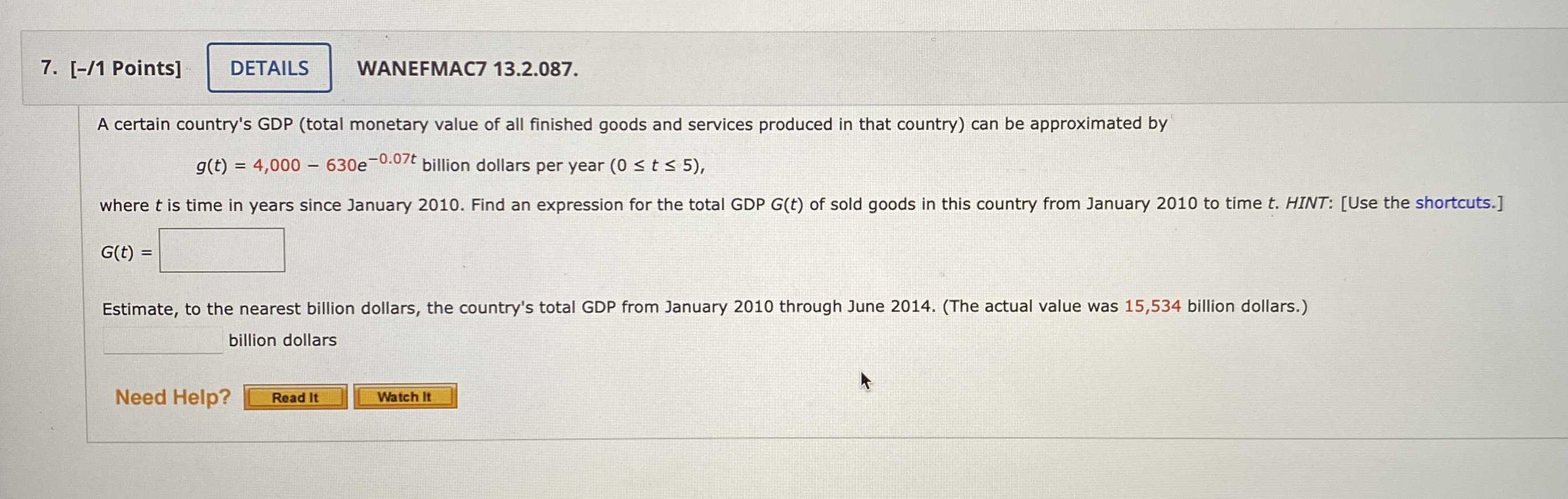### ¿Todavía tienes preguntas de matemáticas?

Pregunte a nuestros tutores expertos
Algebra
PreguntaA certain country's GDP (total monetary value of all finished goods and services produced in that country) can be approximated by $$g ( t ) = 4,000 - 630 e ^ { - 0.07 t }$$ billion dollars per year $$( 0 \leq t \leq 5 )$$ where $$t$$ is time in years since January $$2010$$ . Find an expression for the total GDP G(t) of sold goods in this country from January $$2010$$ to time $$t$$ .

G(t)=$$4000t+ 9000e^{- 0.07t}$$